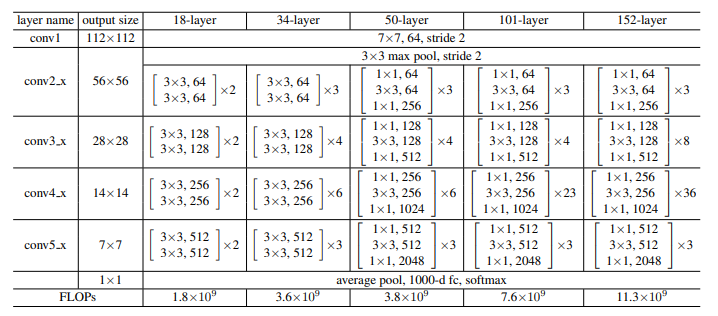# What’s the “Res” in “Resnet”? [Part II]

Source: Deep Learning on Medium# What’s the “Res” in “Resnet”? [Part II]

We really apologize for the delay. We know you have been anxiously waiting for the second part of this series. In the last blog, we saw that degradation leads to poor accuracy in a deeper neural network. In this blog, let us see how they are able to eliminate this problem.

# The Solution

The problem of degradation is addressed by Deep Residual Learning Framework. So what is it all about?

The Deep Residual Learning technique basically proposes the following:

“Instead of hoping a few stacked layers to learn a desired unreferenced mapping x->y denoted by h(x), let a residual function f(x) be defined such that f(x) = h(x) — x, which can be remodified as h(x) = f(x) + x”.

The authors’ hypothesis is that it is easier to optimize the difference f(x) than to optimize the unreferenced mapping h(x). It basically means that if the identity mapping is optimal, it is very easy to make the residual function as 0. In this way, the original experiment of obtaining the same accuracy in a 100-layer network as the 50-layer shallow network was successfully observed.

Types of residual connections:

1. When input and output dimensions are the same: